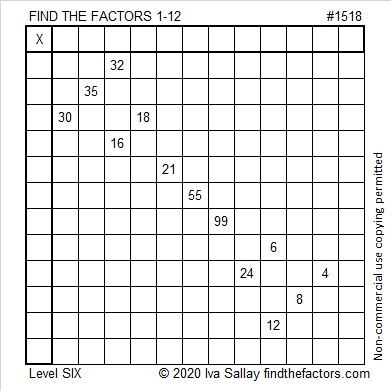1518 and Level 6

Contents

Today’s Puzzle:

Level 6 puzzles are designed to be a little tricky. Just make sure you use logic to figure out the factors every time, and you will get it done!Factors of 1518:

• 1518 is a composite number.
• Prime factorization: 1518 = 2 × 3 × 11 × 23.
• 1518 has no exponents greater than 1 in its prime factorization, so √1518 cannot be simplified.
• The exponents in the prime factorization are 1, 1, 1, and 1. Adding one to each exponent and multiplying we get (1 + 1)(1 + 1)(1 + 1)(1 + 1) = 2 × 2 × 2 × 2 = 16. Therefore 1518 has exactly 16 factors.
• The factors of 1518 are outlined with their factor pair partners in the graphic below.One More Fact about the Number 1518:

1518 has a palindromic prime factorization. (The digits are the same frontward or backward.)
1518 = 2 · 3 · 11 · 23

This site uses Akismet to reduce spam. Learn how your comment data is processed.